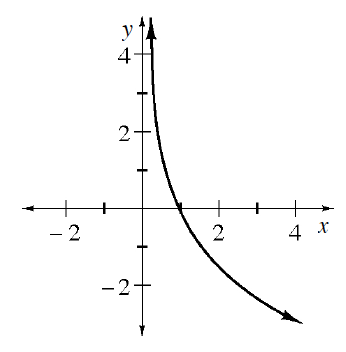### Home > CCA2 > Chapter 6 > Lesson 6.2.1 > Problem6-98

6-98.

Below is a graph of $y=\log_bx$. Describe the possible values for $b$.Think of the graph of $\log(x)$. How is this graph different?
How would that affect the equation?
What is the maximum that $b$ could be? What is the minimum?

Use the eTool below to see how different values of b change the graph.
Click the link at right for the full version of the eTool: 6-98 HW eTool